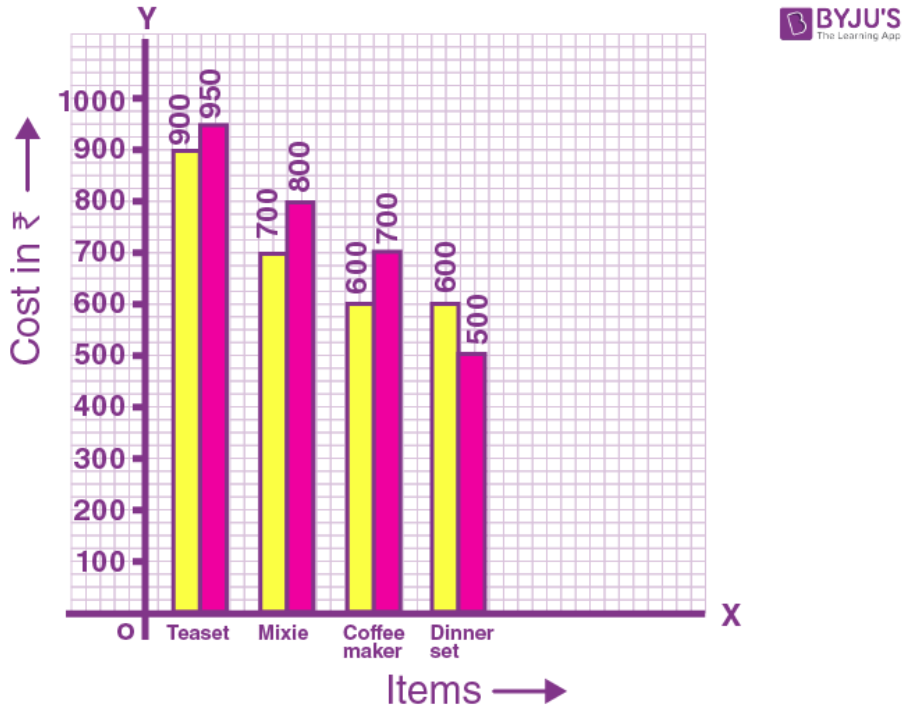# Selina Solutions Concise Maths Class 8 Chapter 22: Data Handling

Selina Solutions Concise Maths Class 8 Chapter 22 Data Handling is a very important chapter as it creates an awareness among students on how to handle the large data given to them at a time. The faculty at BYJU’S have designed the solutions as per the syllabus prescribed for the academic year. The solutions are presented in a step wise manner with brief explanations in order to enhance the conceptual learning among students. By solving the problems using the ICSE Selina Solutions Class 8 Maths Chapter 22 Data Handling PDF, students will be able to obtain a good score in the annual exam.

From this chapter, students will be able to understand the method of handling the large data given to them in a simple and systematic way. It not only provides students with knowledge about the concepts covered in the textbook but also makes them aware of the applications in our day to day lives. Students under the ICSE Class 8 are advised to refer to the Selina Solutions while solving the textbook questions to get their doubts cleared instantly.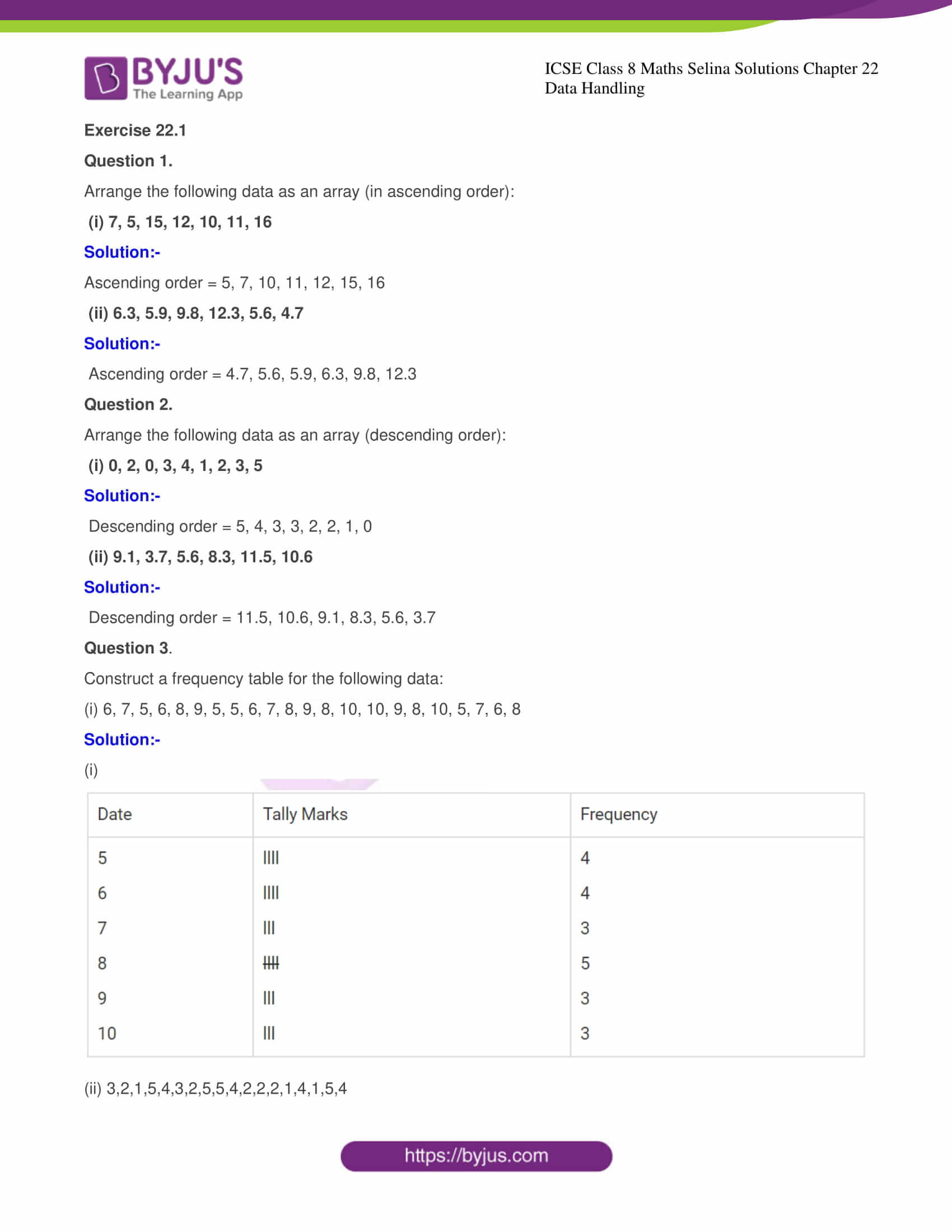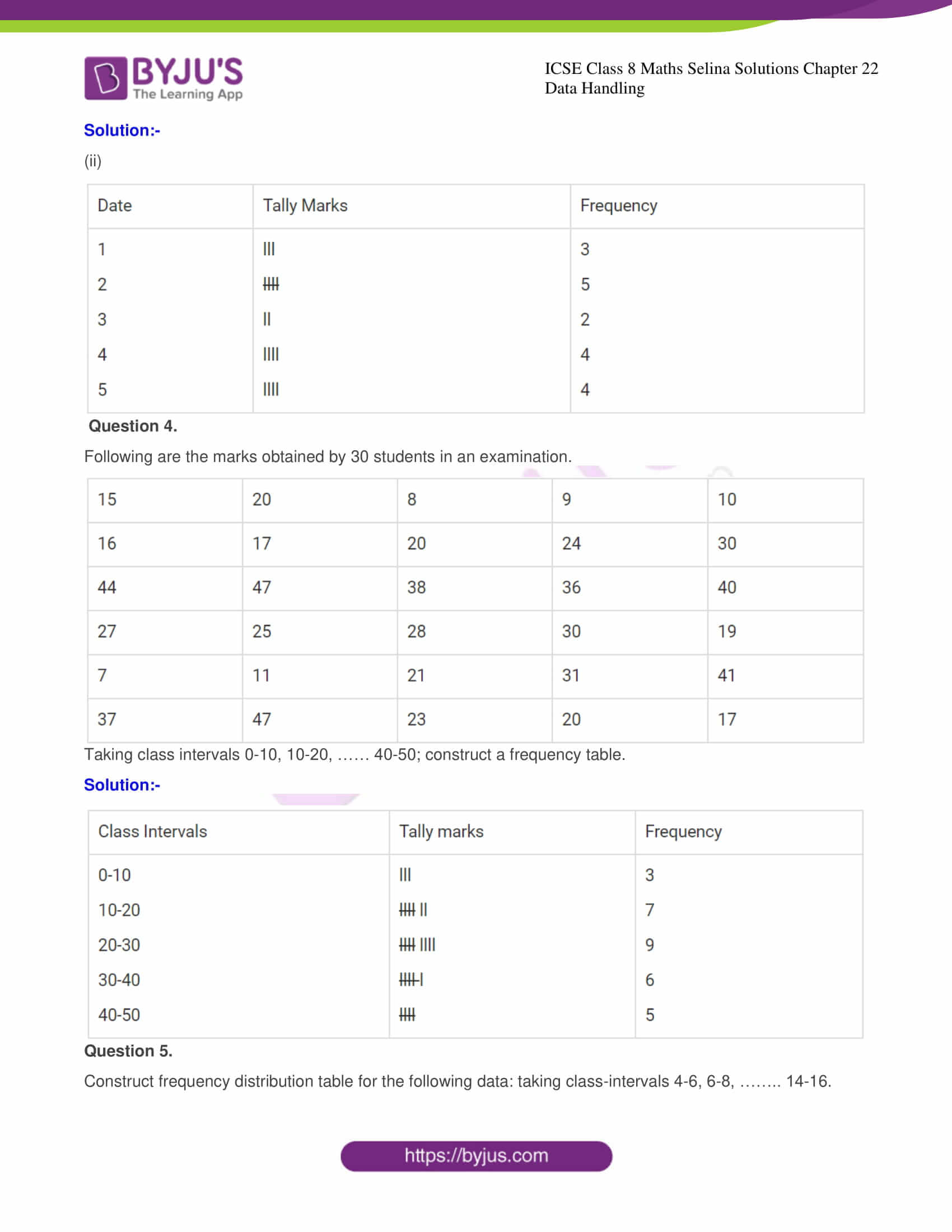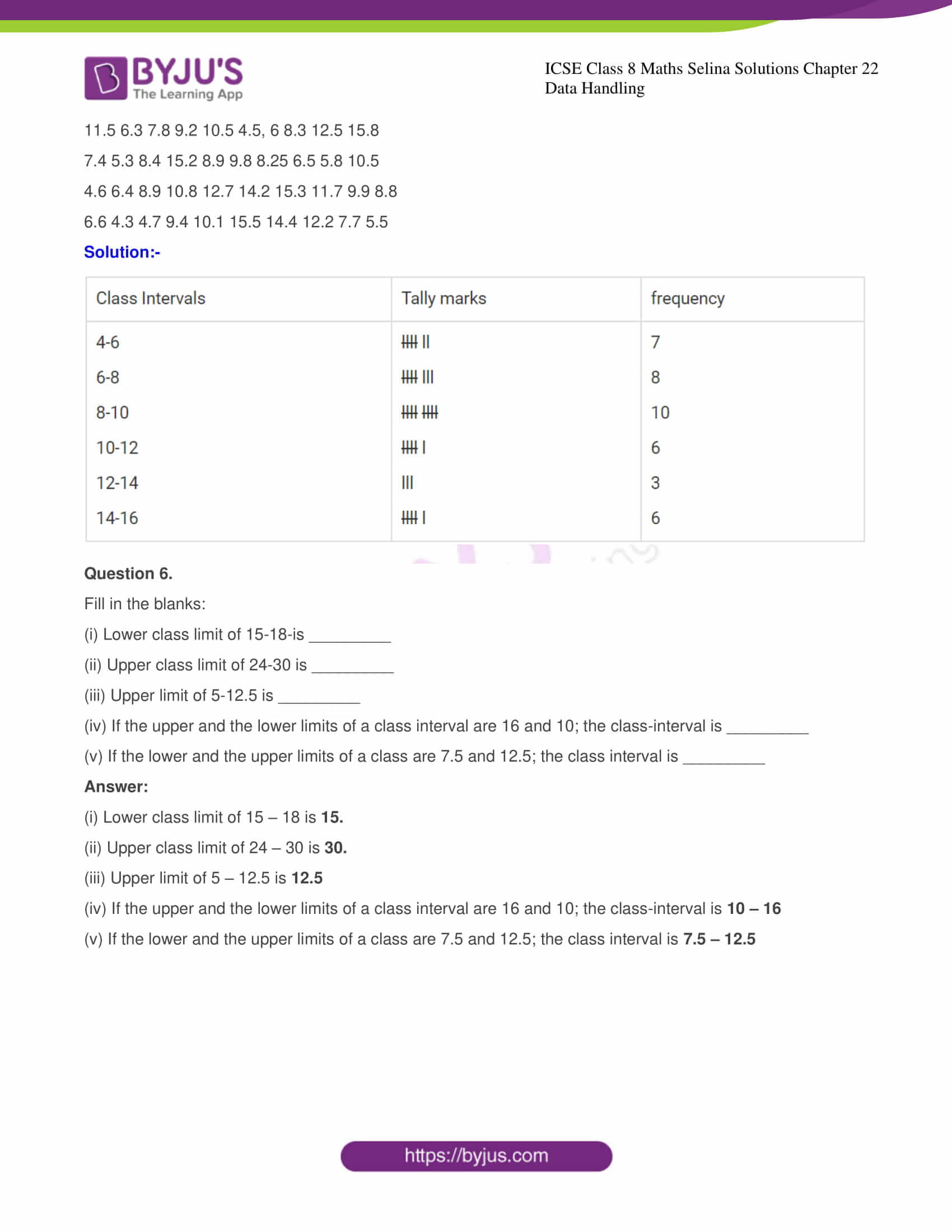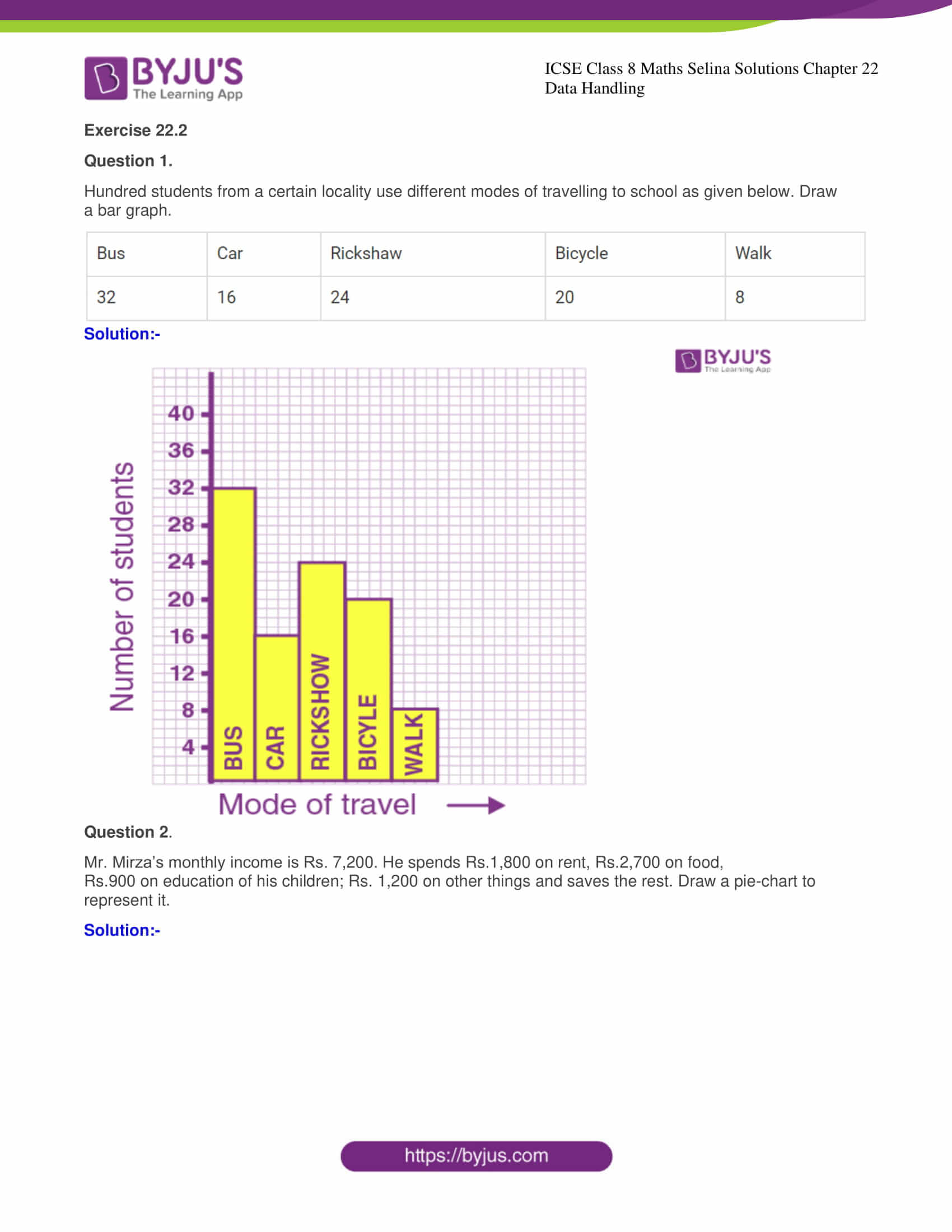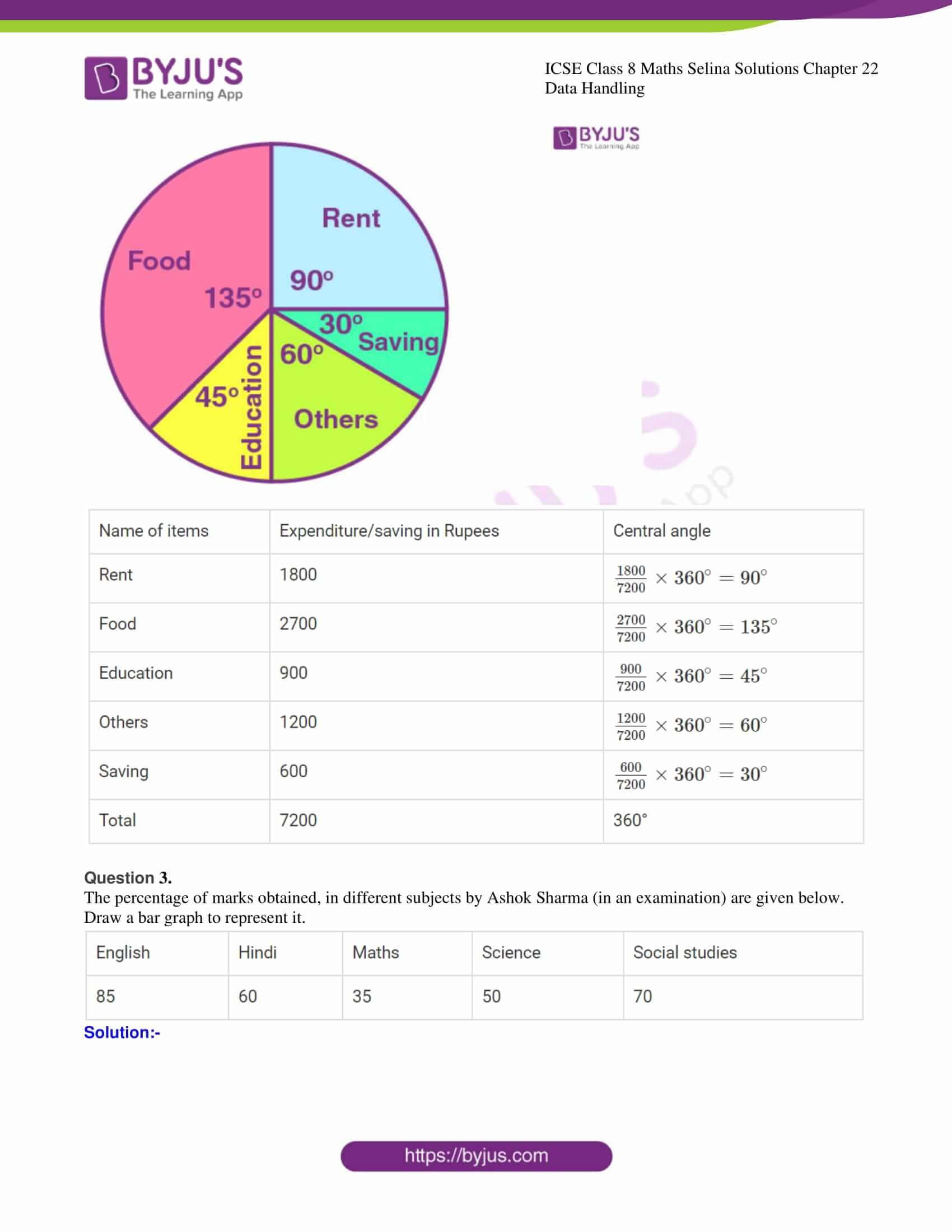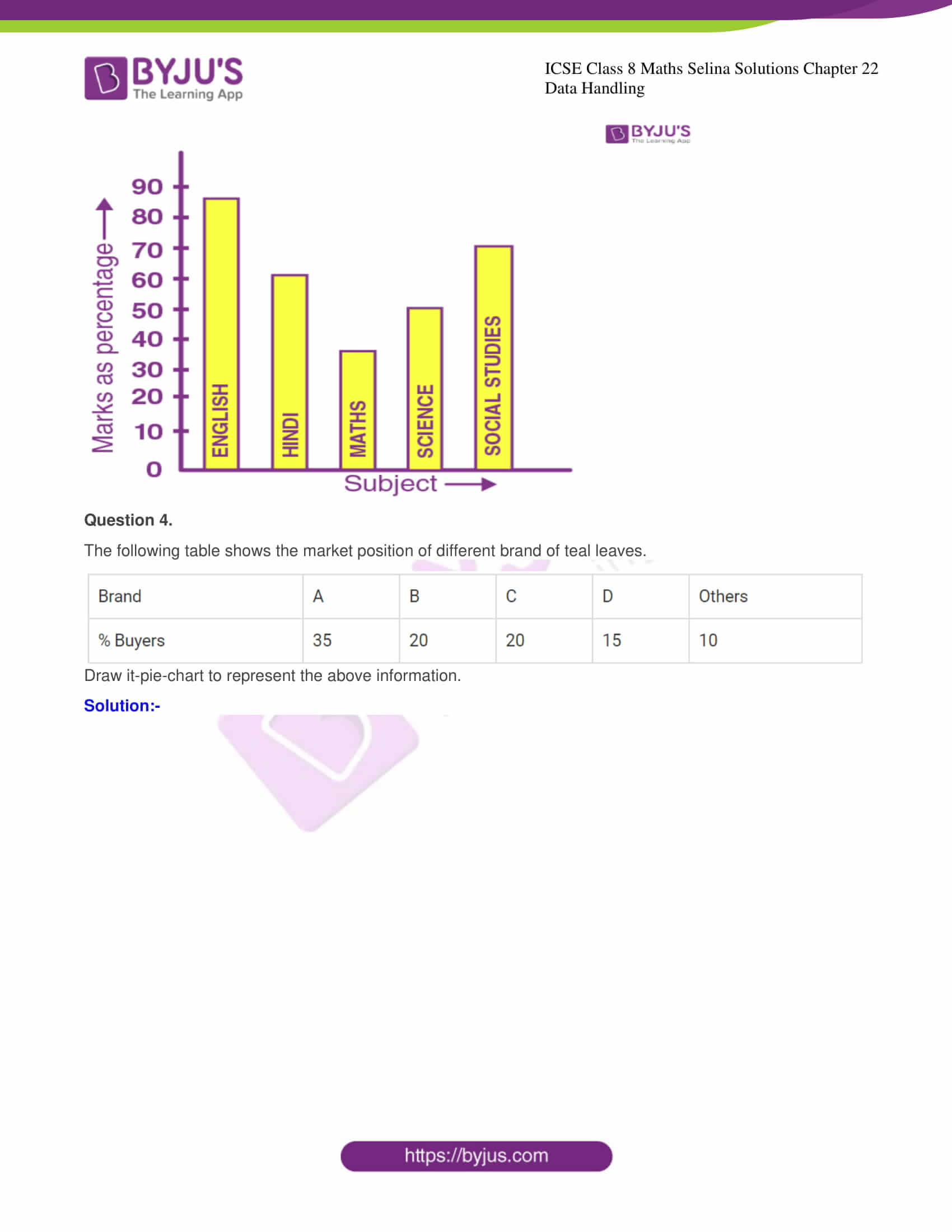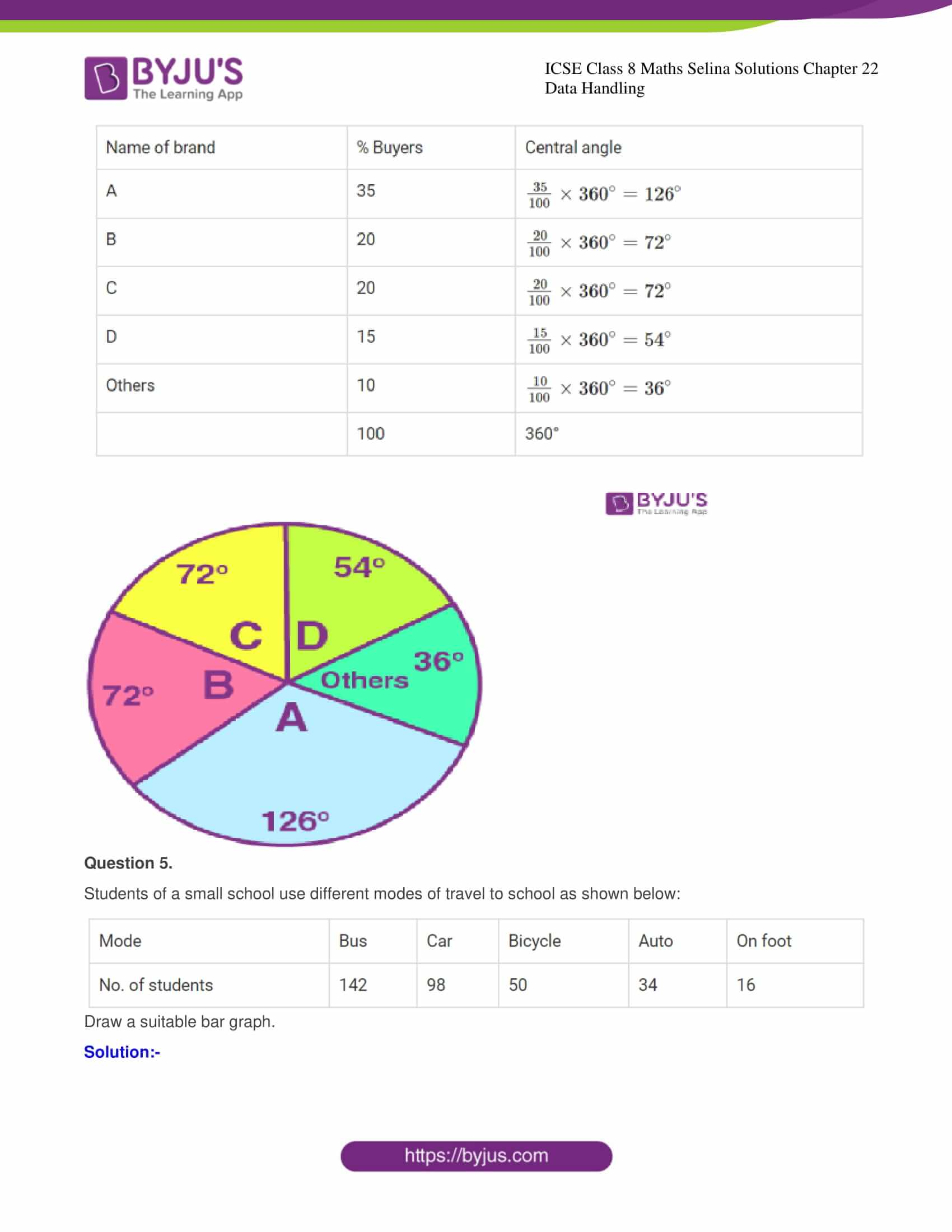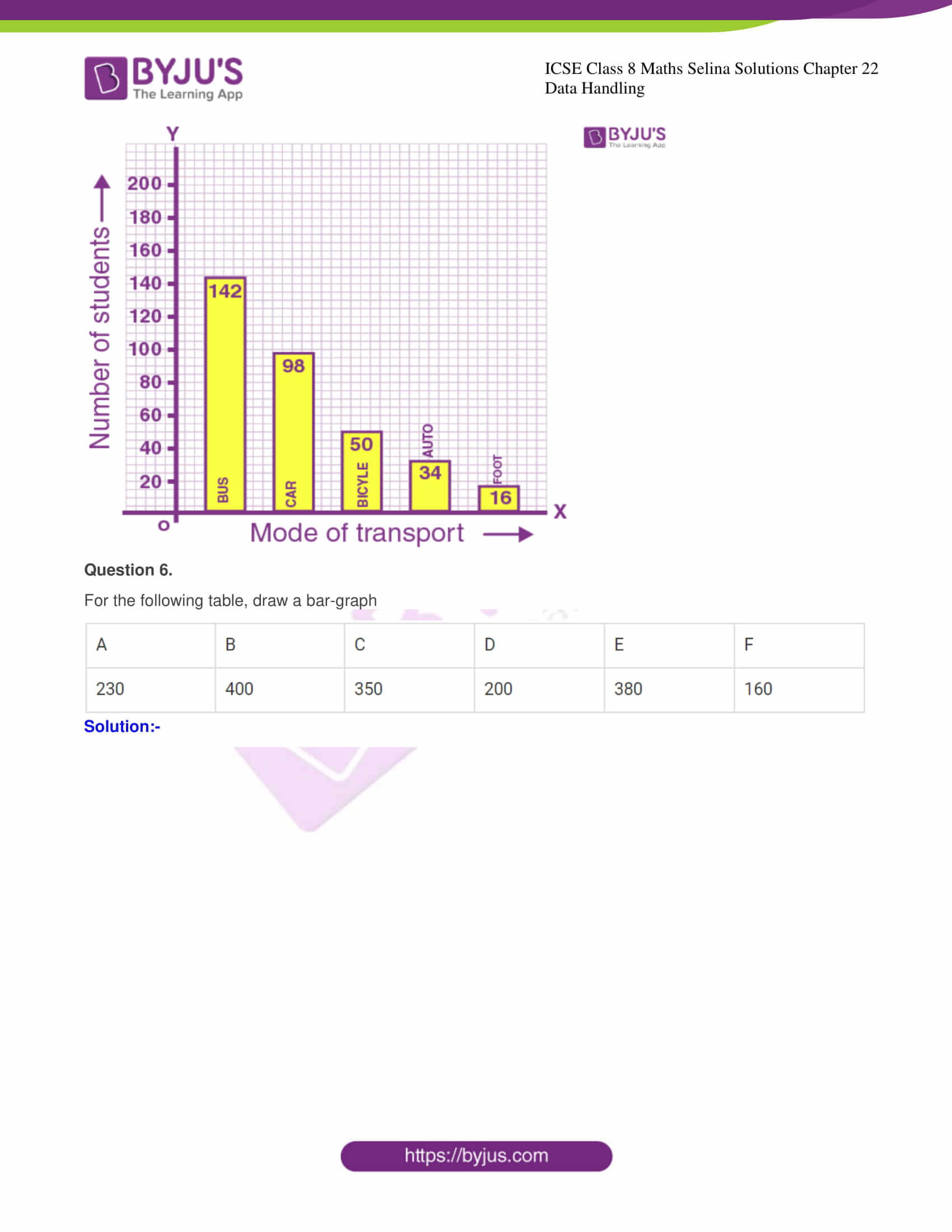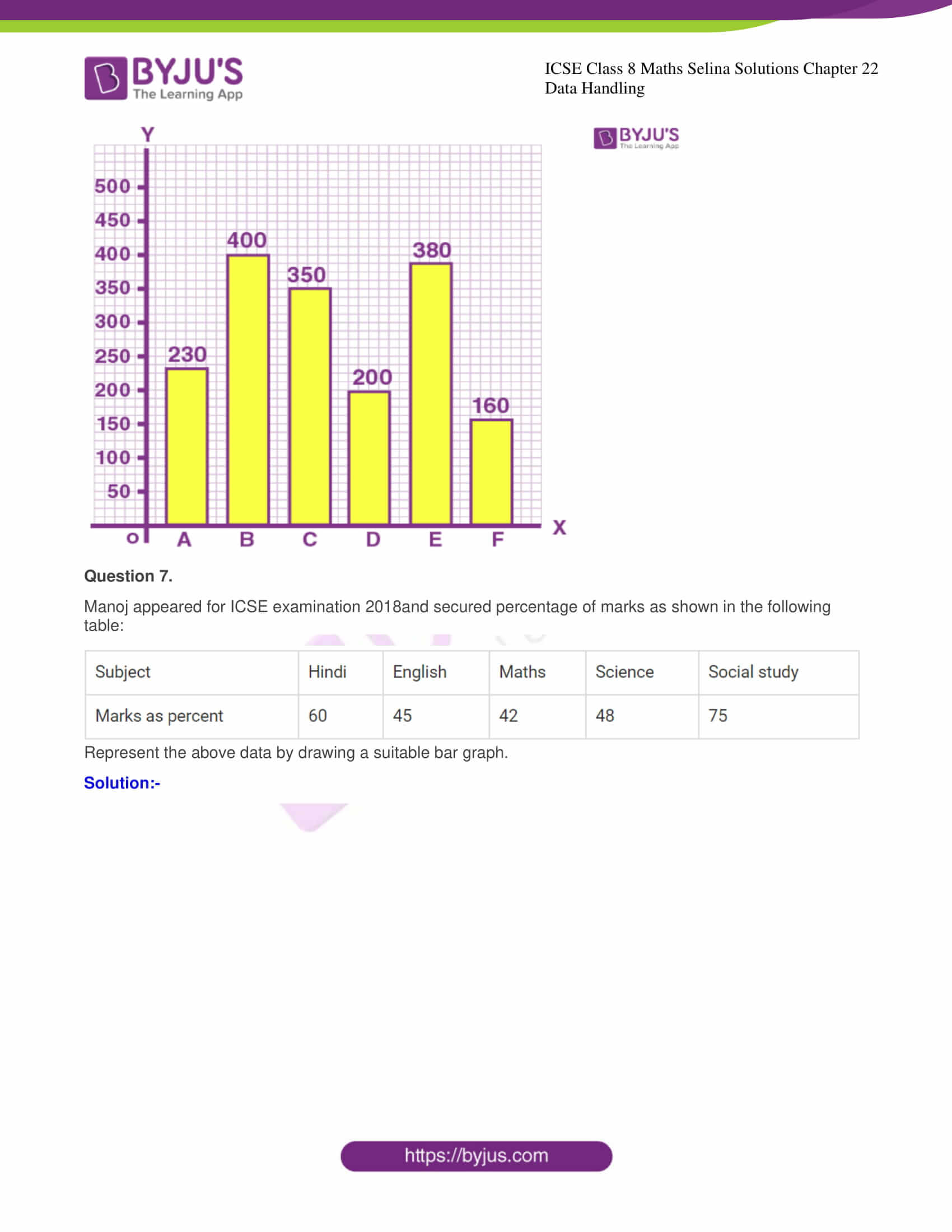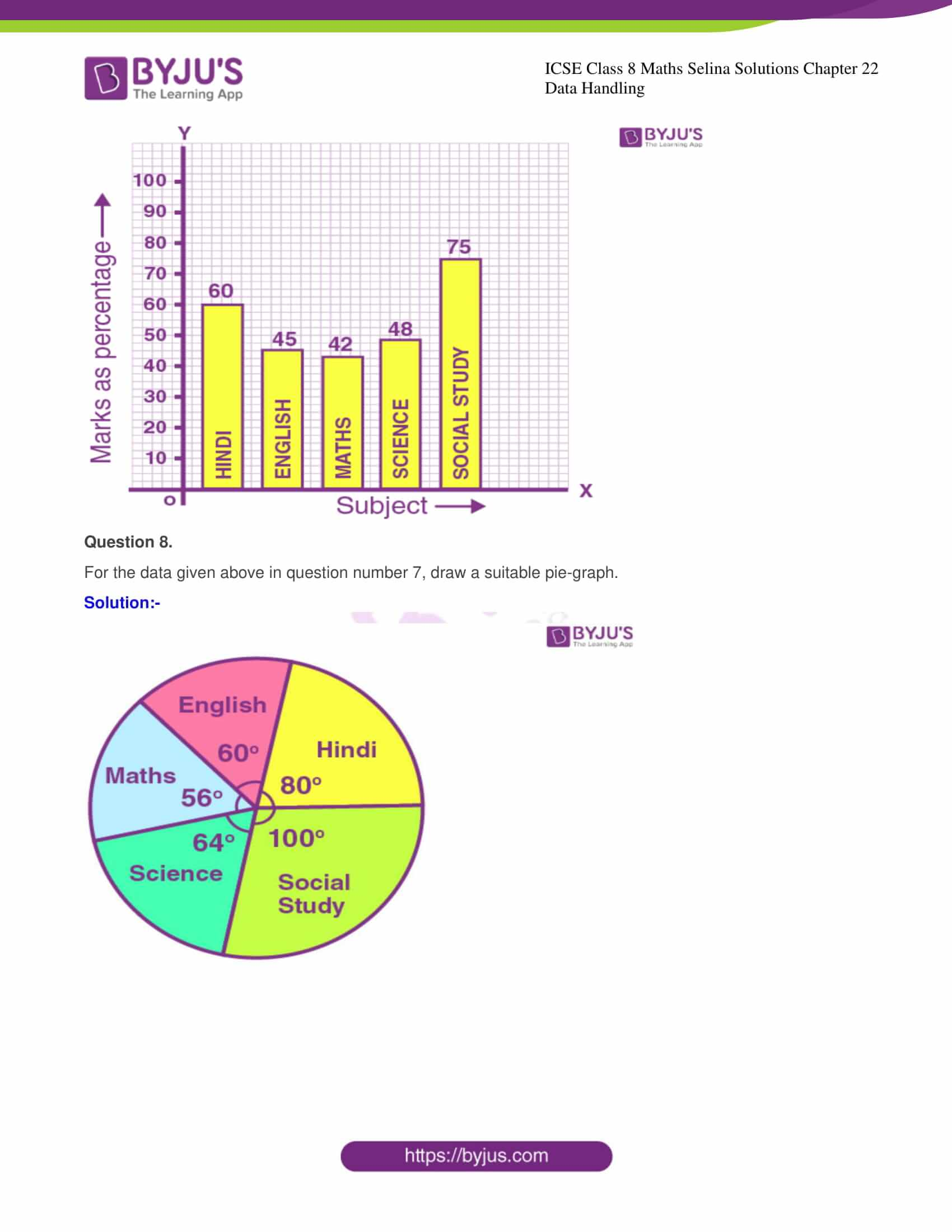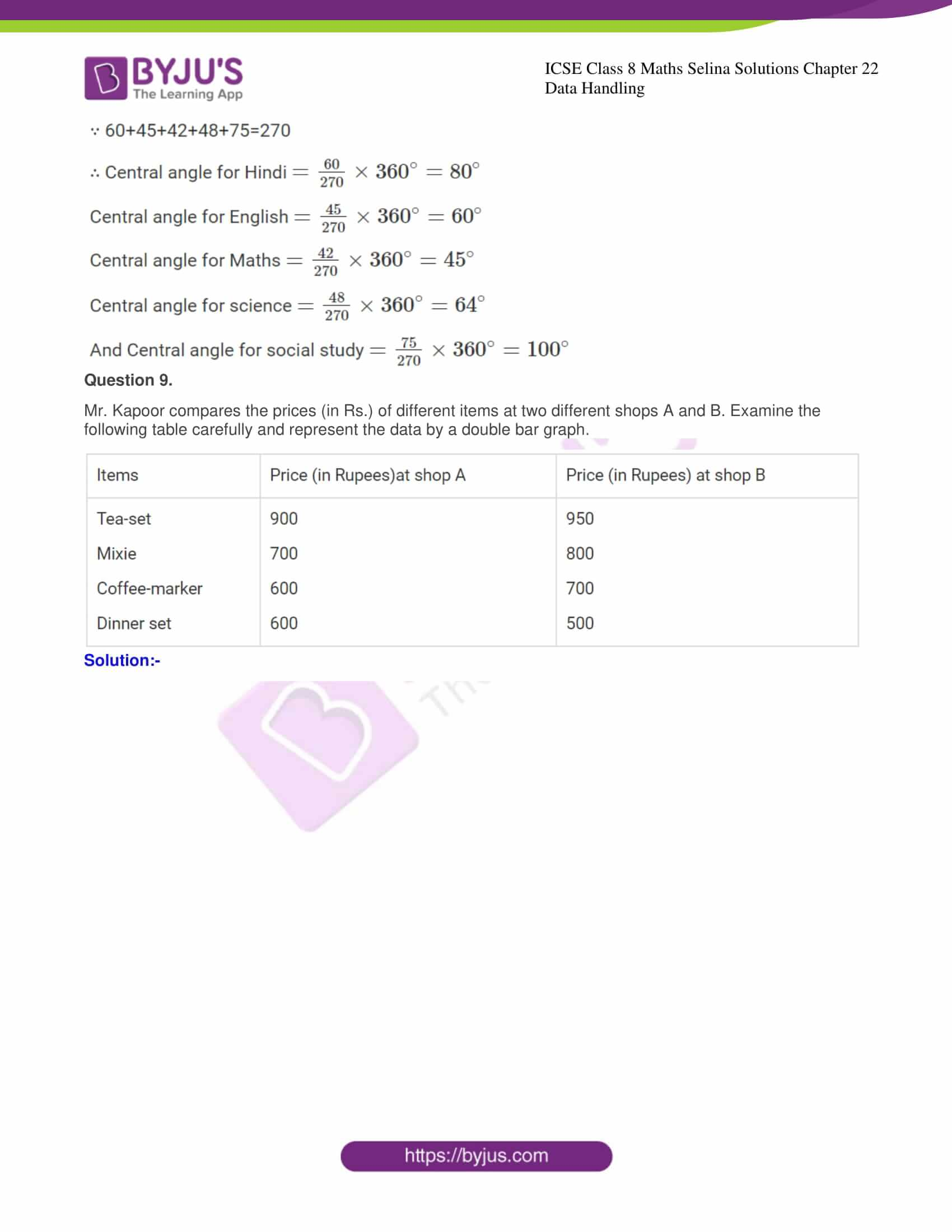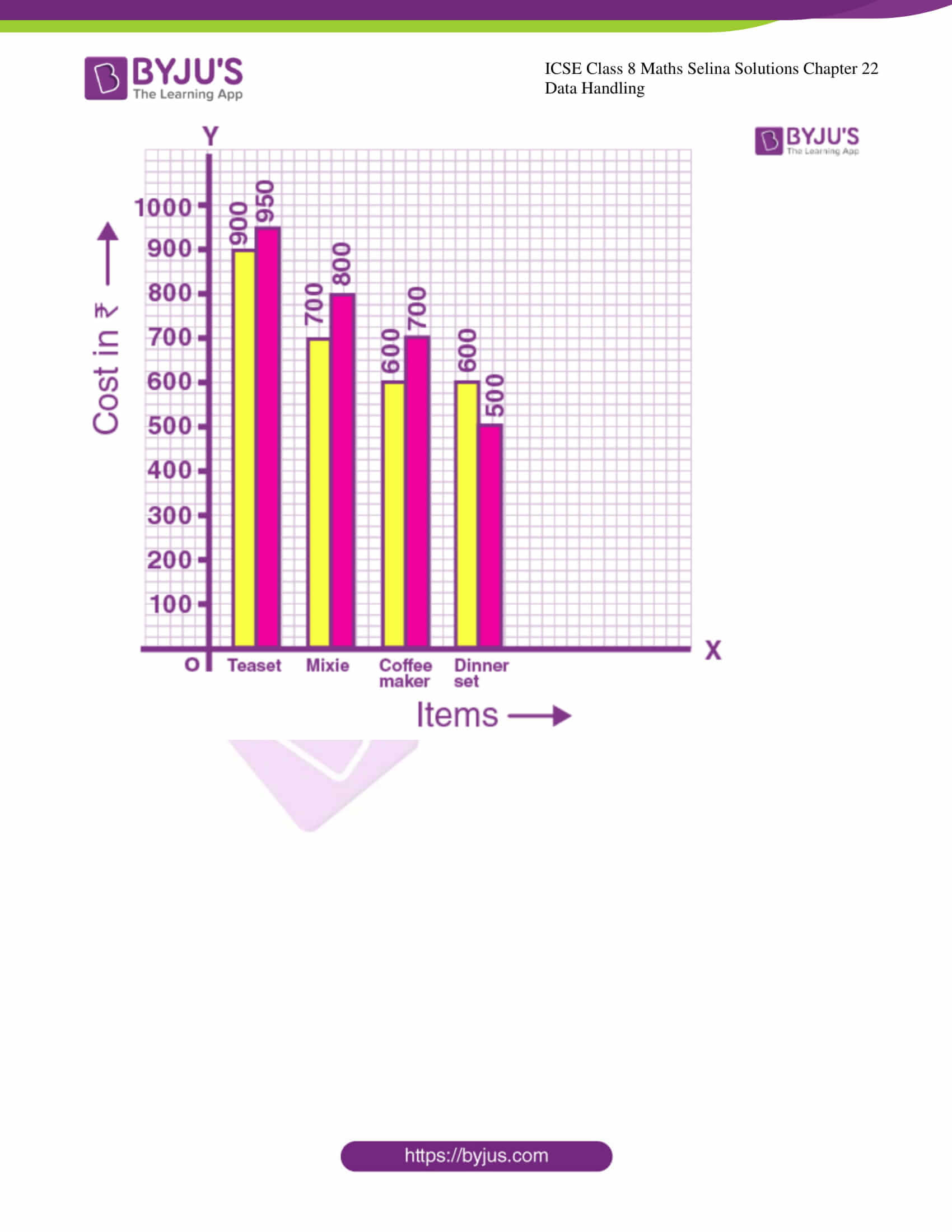## Access Selina Solutions Concise Maths Class 8 Chapter 22: Data Handling

Exercise 22.1

Question 1.

Arrange the following data as an array (in ascending order):

(i) 7, 5, 15, 12, 10, 11, 16

Solution:-

Ascending order = 5, 7, 10, 11, 12, 15, 16

(ii) 6.3, 5.9, 9.8, 12.3, 5.6, 4.7

Solution:-

Ascending order = 4.7, 5.6, 5.9, 6.3, 9.8, 12.3

Question 2.

Arrange the following data as an array (descending order):

(i) 0, 2, 0, 3, 4, 1, 2, 3, 5

Solution:-

Descending order = 5, 4, 3, 3, 2, 2, 1, 0

(ii) 9.1, 3.7, 5.6, 8.3, 11.5, 10.6

Solution:-

Descending order = 11.5, 10.6, 9.1, 8.3, 5.6, 3.7

Question 3.

Construct a frequency table for the following data:

(i) 6, 7, 5, 6, 8, 9, 5, 5, 6, 7, 8, 9, 8, 10, 10, 9, 8, 10, 5, 7, 6, 8

Solution:-

(i)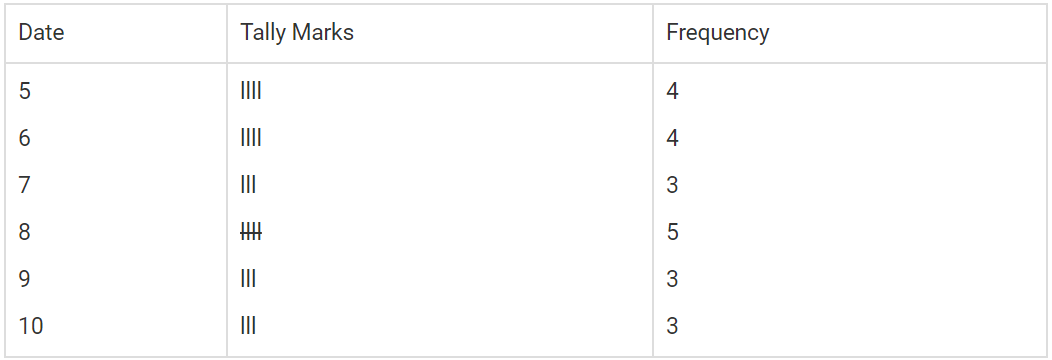(ii) 3,2,1,5,4,3,2,5,5,4,2,2,2,1,4,1,5,4

Solution:-

(ii)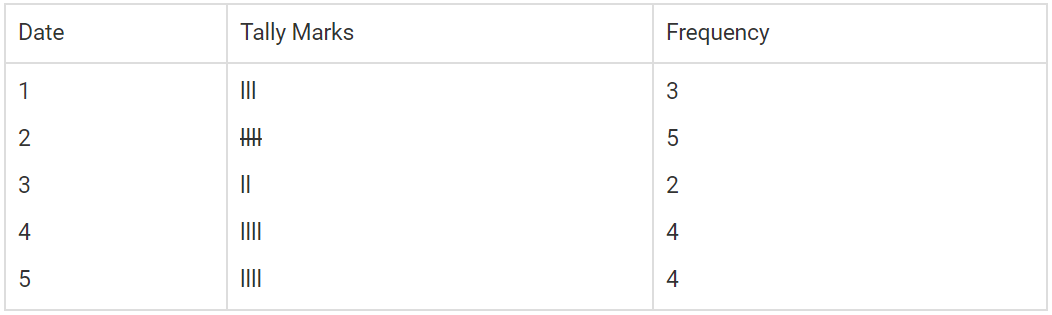Question 4.

Following are the marks obtained by 30 students in an examination.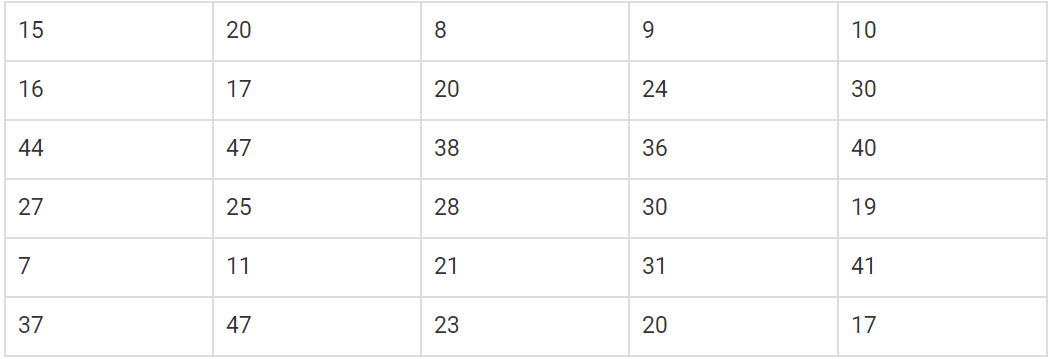Taking class intervals 0-10, 10-20, …… 40-50; construct a frequency table.

Solution:-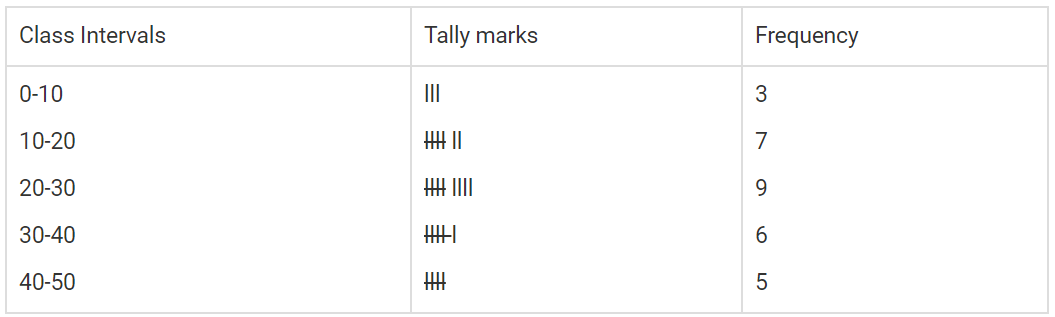Question 5.

Construct frequency distribution table for the following data: taking class-intervals 4-6, 6-8, …….. 14-16.

11.5 6.3 7.8 9.2 10.5 4.5, 6 8.3 12.5 15.8

7.4 5.3 8.4 15.2 8.9 9.8 8.25 6.5 5.8 10.5

4.6 6.4 8.9 10.8 12.7 14.2 15.3 11.7 9.9 8.8

6.6 4.3 4.7 9.4 10.1 15.5 14.4 12.2 7.7 5.5

Solution:-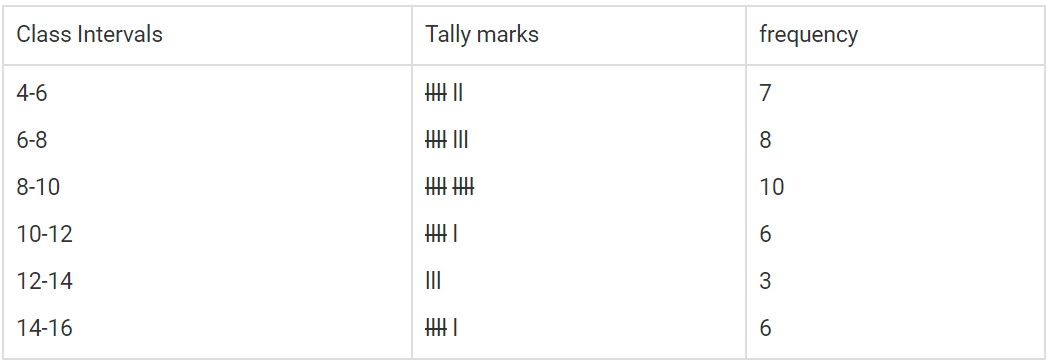Question 6.

Fill in the blanks:

(i) Lower class limit of 15-18-is _________

(ii) Upper class limit of 24-30 is _________

(iii) Upper limit of 5-12.5 is _________

(iv) If the upper and the lower limits of a class interval are 16 and 10; the class-interval is _________

(v) If the lower and the upper limits of a class are 7.5 and 12.5; the class interval is _________

(i) Lower class limit of 15 – 18 is 15.

(ii) Upper class limit of 24 – 30 is 30.

(iii) Upper limit of 5 – 12.5 is 12.5

(iv) If the upper and the lower limits of a class interval are 16 and 10; the class-interval is 10 – 16

(v) If the lower and the upper limits of a class are 7.5 and 12.5; the class interval is 7.5 – 12.5

Exercise 22.2

Question 1.

Hundred students from a certain locality use different modes of travelling to school as given below. Draw a bar graph.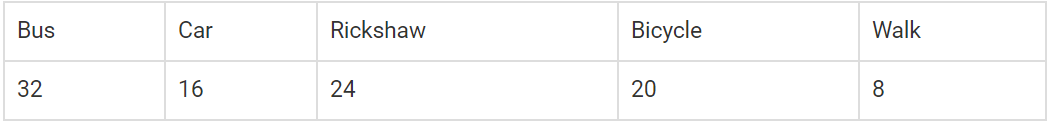Solution:-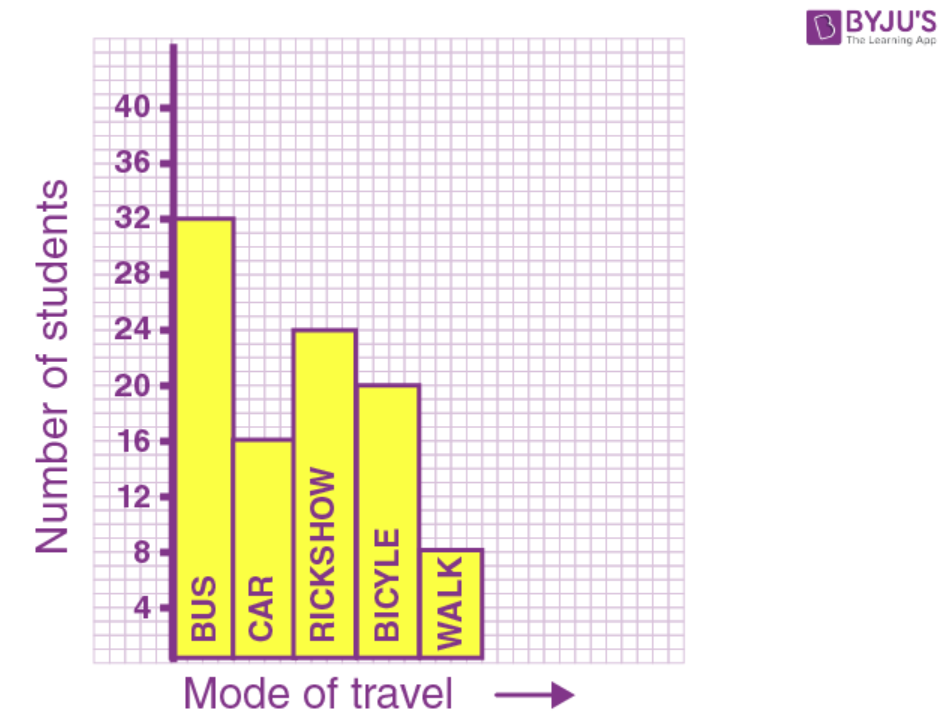Question 2.

Mr. Mirza’s monthly income is Rs. 7,200. He spends Rs.1,800 on rent, Rs.2,700 on food, Rs.900 on education of his children; Rs. 1,200 on other things and saves the rest. Draw a pie-chart to represent it.

Solution:-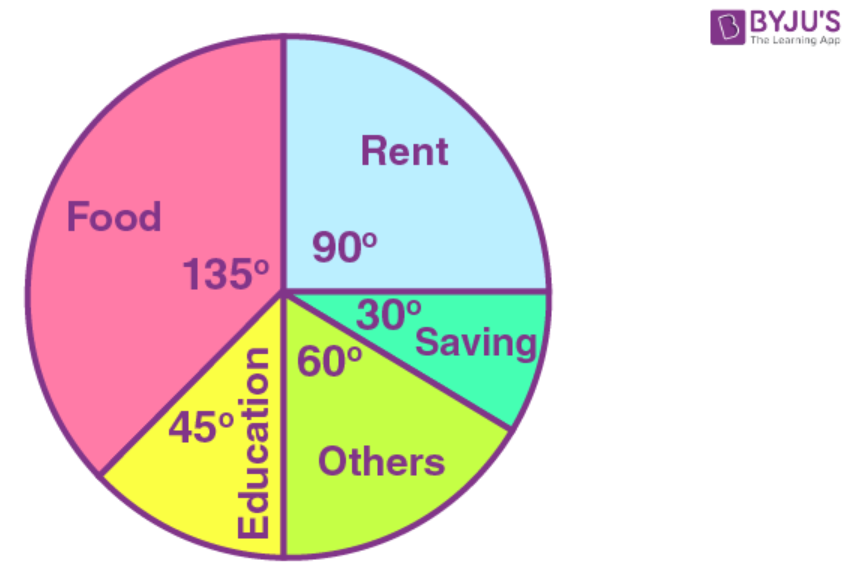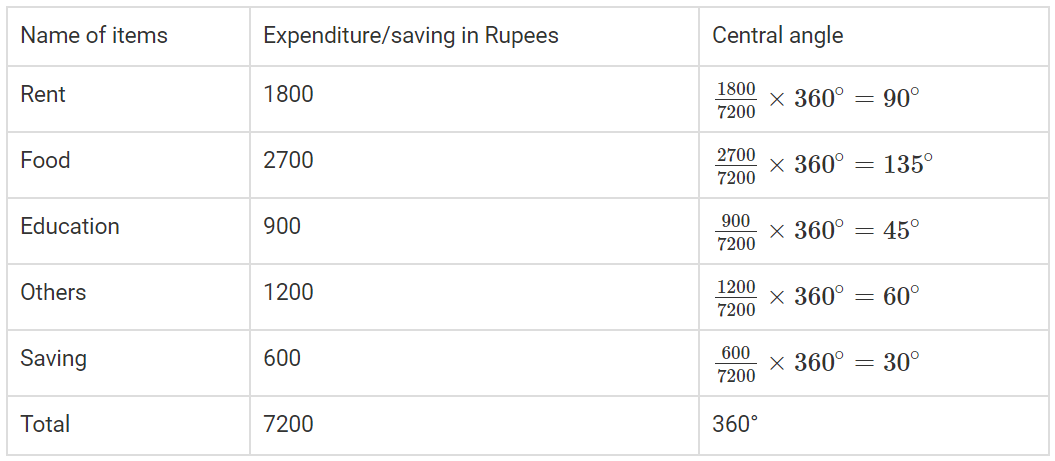Question 3. The percentage of marks obtained, in different subjects by Ashok Sharma (in an examination) are given below.

Draw a bar graph to represent it.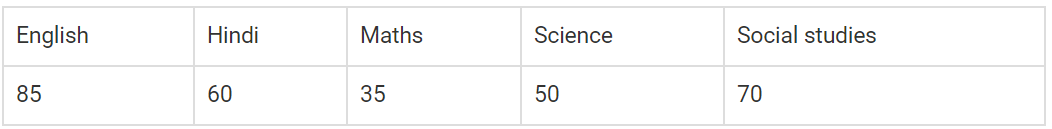Solution:-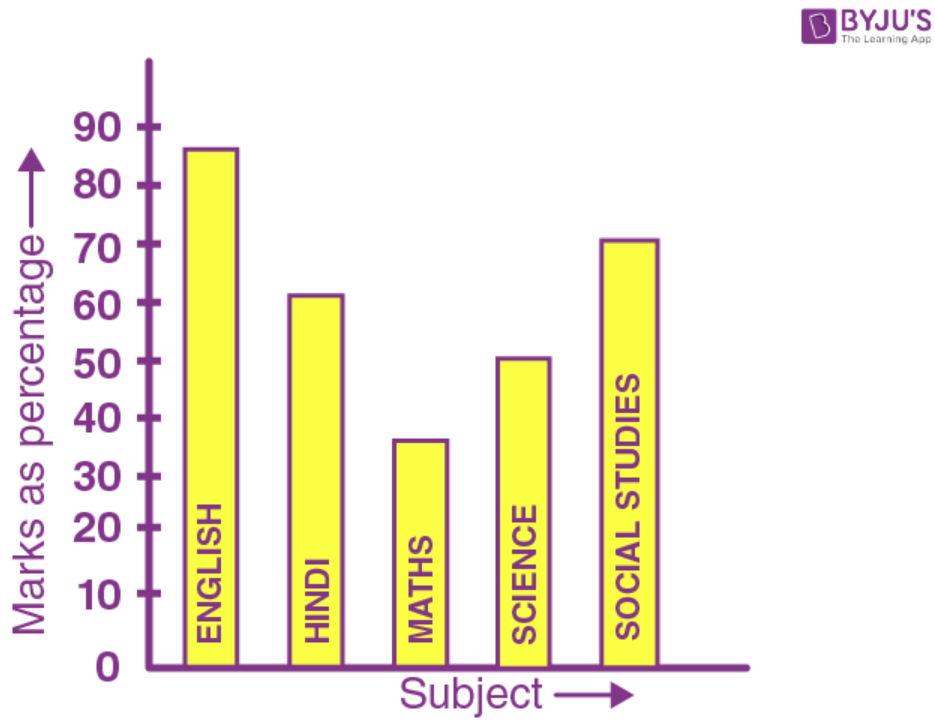Question 4.

The following table shows the market position of different brand of teal leaves.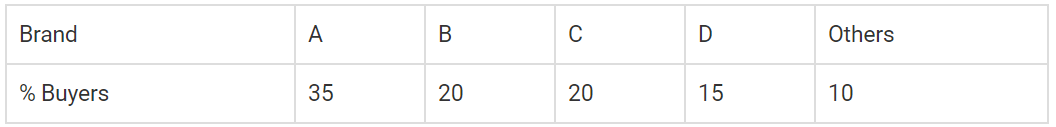Draw it-pie-chart to represent the above information.

Solution:-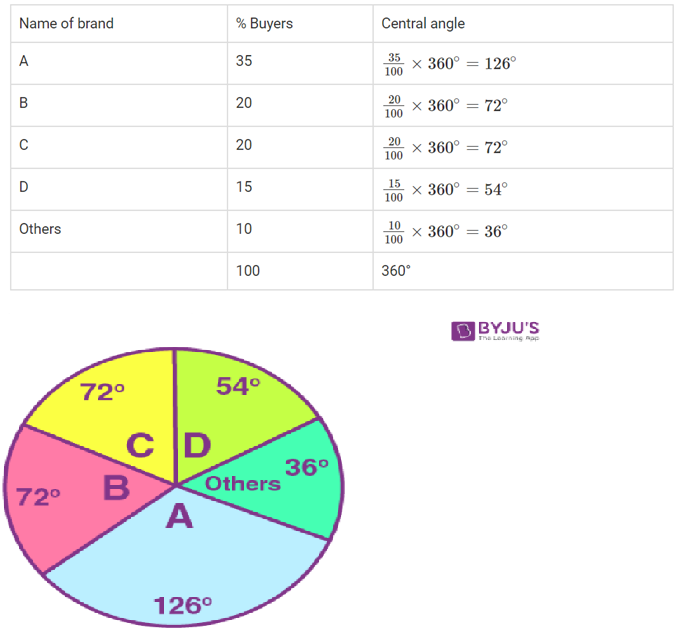Question 5.

Students of a small school use different modes of travel to school as shown below: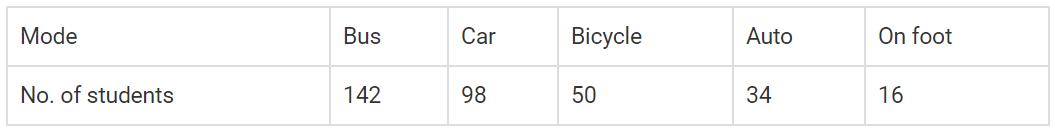Draw a suitable bar graph.

Solution:-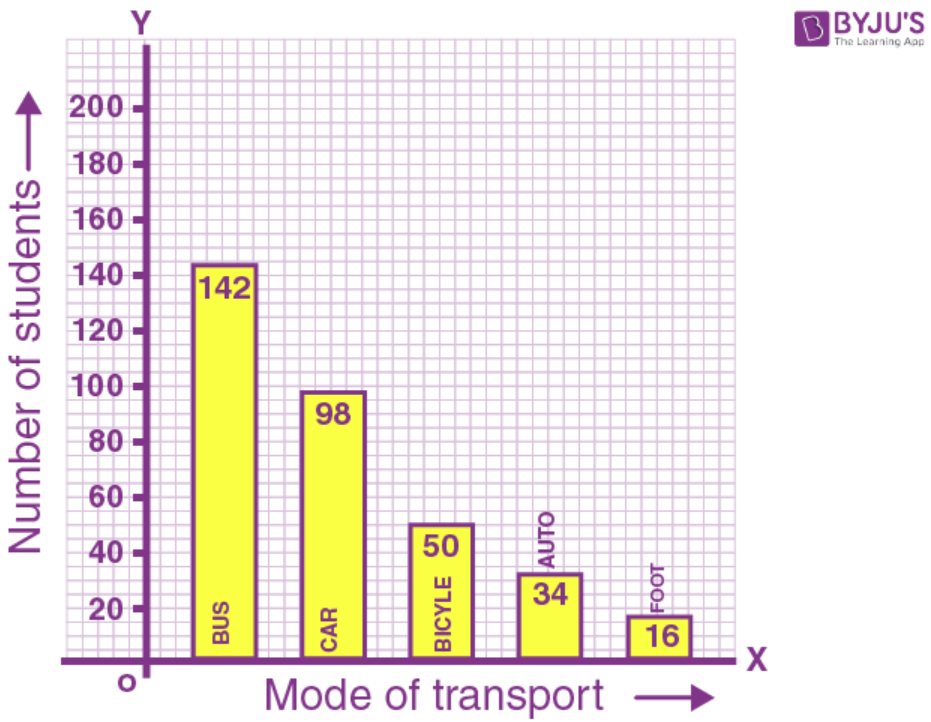Question 6.

For the following table, draw a bar-graph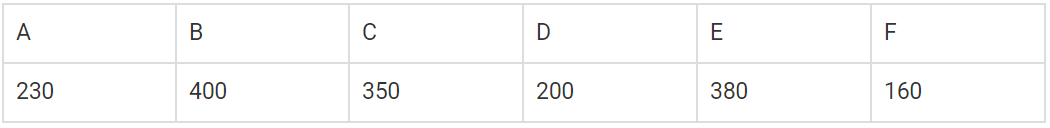Solution:-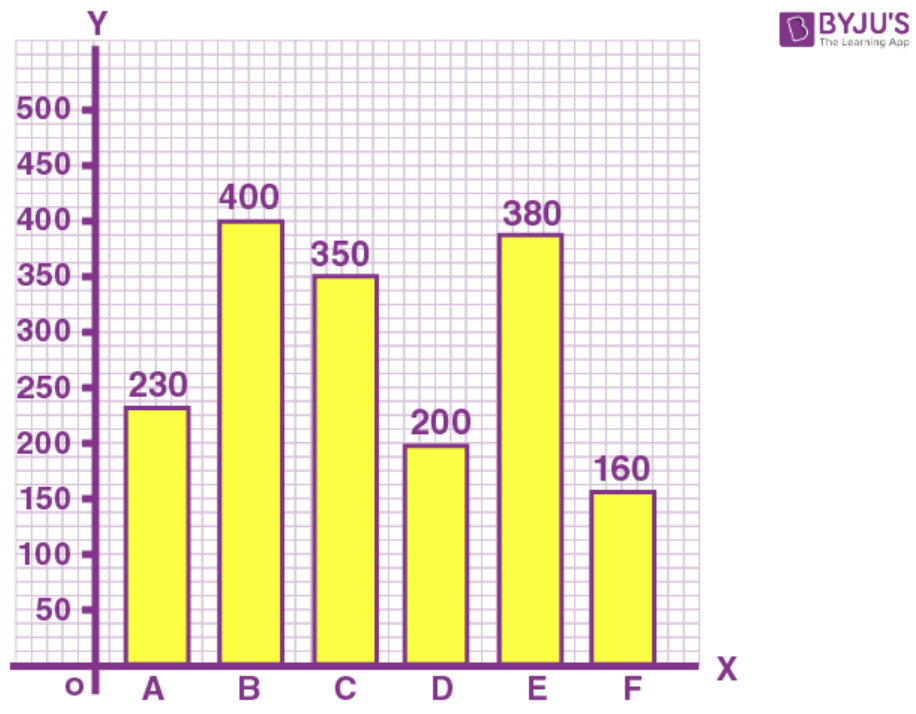Question 7.

Manoj appeared for ICSE examination 2018and secured percentage of marks as shown in the following table: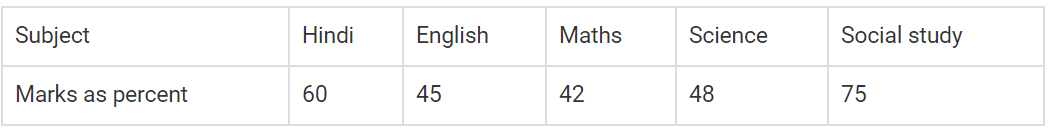Represent the above data by drawing a suitable bar graph.

Solution:-Question 8.

For the data given above in question number 7, draw a suitable pie-graph.

Solution:-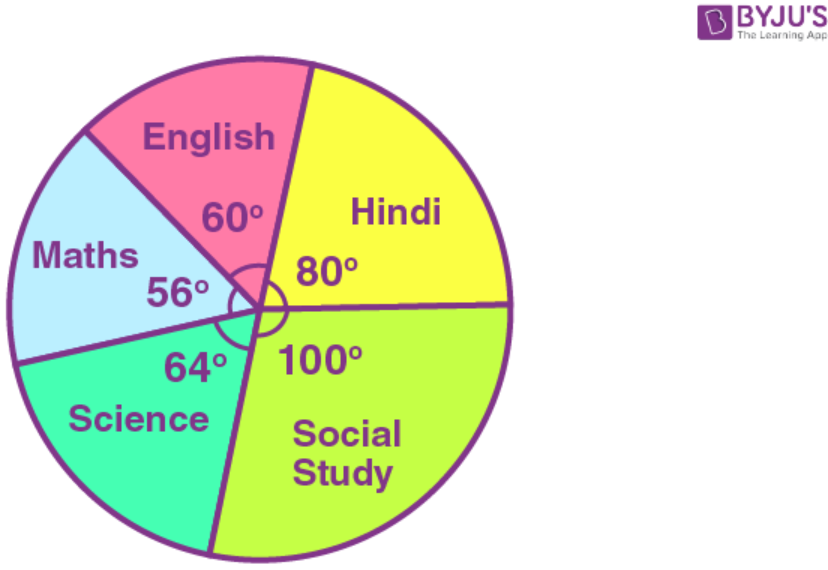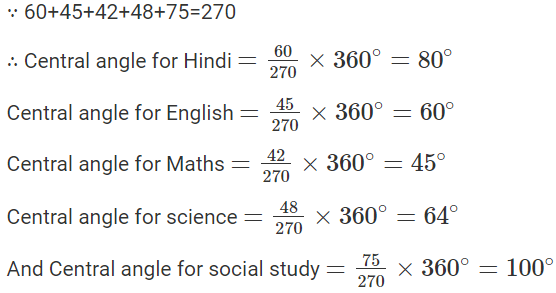Question 9.

Mr. Kapoor compares the prices (in Rs.) of different items at two different shops A and B. Examine the following table carefully and represent the data by a double bar graph.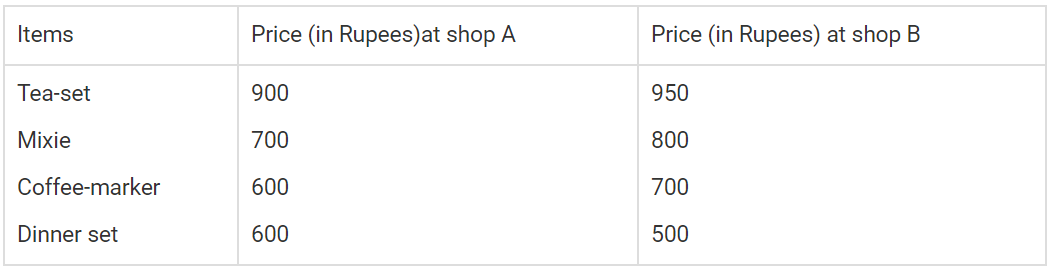Solution:-